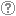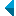# Search the repositoryQuery: search in TitleAuthorAbstractKeywordsFull textYear of publishing ANDORAND NOT search in TitleAuthorAbstractKeywordsFull textYear of publishing ANDORAND NOT search in TitleAuthorAbstractKeywordsFull textYear of publishing ANDORAND NOT search in TitleAuthorAbstractKeywordsFull textYear of publishing Work type: All work types Habilitation (m4) Specialist thesis (m3) High school thesis (m6) Bachelor work * (dip) Master disertations * (mag) Doctorate disertations * (dok) Research Data or Corpuses (data) * old and bologna study programme Language: All languagesSlovenianEnglishGermanCroatianSerbianBosnianBulgarianCzechFinnishFrenchGerman (Austria)HungarianItalianJapaneseLithuanianNorwegianPolishRussianSerbian (cyrillic)SlovakSpanishSwedishTurkishUnknown Search in: RUP    FAMNIT - Faculty of Mathematics, Science and Information Technologies    FHŠ - Faculty of Humanities    FM - Faculty of Management    FTŠ Turistica - Turistica – College of Tourism Portorož    FVZ - Faculty of Health Sciences    IAM - Andrej Marušič Institute    PEF - Faculty of Education    UPR - University of Primorska    ZUP - University of Primorska PressCOBISS    Fakulteta za humanistične študije, Koper    Fakulteta za management Koper in Pedagoška fakulteta Koper    Fakulteta za vede o zdravju, Izola    Knjižnica za tehniko, medicino in naravoslovje, Koper    Turistica, Portorož    Znanstveno-raziskovalno središče Koper Options: Show only hits with full text Reset

 1 - 10 / 1011.Cao, Chongguang; Huang, Liping; Tang, Xiaomin: Additive map preserving rank 2 on alternate matrices. (English). - [J] Afr. Diaspora J. Math 3, No. 2, 107-113 (2005). [ISSN 1539-845X]Bojan Kuzma, 2006, review, book review, critiqueFound in: ključnih besedahSummary of found: ...matematika, linear algebra, aditivni ohranjevalec, rang, ...Keywords: matematika, linear algebra, aditivni ohranjevalec, rangPublished: 15.10.2013; Views: 2700; Downloads: 22Full text (0,00 KB) 2.Jordan triple product homomorphismsBojan Kuzma, 2006, original scientific articleAbstract: Nondegenerate mappings that preserve Jordan triple product on ▫${\mathscr{M}}_n({\mathbb{F}}$▫ are characterized. Here, ▫$n \ge 3$▫ and ▫$\mathbb{F}$▫ is an arbitrary field.Found in: ključnih besedahSummary of found: ...mathematics, linear algebra, matrix algebra, Jordan triple product, nonlinear...Keywords: mathematics, linear algebra, matrix algebra, Jordan triple product, nonlinear preserverPublished: 15.10.2013; Views: 3236; Downloads: 134Full text (0,00 KB) 3.Noninvertibility preservers on Banach algebrasBojan Kuzma, 2006, short scientific articleAbstract: It is proved that a linear surjection ▫$\Phi: \mathcal{A} \to \mathcal{B}$▫, which preserves noninvertibility between semisimple, unital, complex Banach algebras, is automatically injective.Found in: ključnih besedahSummary of found: ...It is proved that a linear surjection ▫$\Phi: \mathcal{A} \to \mathcal{B}$▫, which preserves... ...analysis, linear preserver, noninvertible element, semisimple Banach algebra, socle...Keywords: mathematics, functional analysis, linear preserver, noninvertible element, semisimple Banach algebra, soclePublished: 15.10.2013; Views: 2368; Downloads: 120Full text (0,00 KB) 4.General preservers of quasi-commutativity on hermitian matricesGregor Dolinar, Bojan Kuzma, 2008, original scientific articleAbstract: Let ▫$H_n$▫ be the set of all ▫$n \times n$▫ hermitian matrices over ▫$\mathbb{C}$▫, ▫$n \ge 3$▫. It is said that ▫$A,B \in H_n$▫ quasi-commute if there exists a nonzero ▫$\xi \in \mathbb{C}$▫ such that ▫$AB = \xi BA$▫ Bijective not necessarily linear maps on hermitian matrices which preserve quasi-commutativity in both directions are classified.Found in: ključnih besedahSummary of found: ...▫$AB = \xi BA$▫ Bijective not necessarily linear maps on hermitian matrices which preserve quasi-commutativity... ...mathematics, linear algebra, general preserver, hermitian matrices, quasi-commutativity...Keywords: mathematics, linear algebra, general preserver, hermitian matrices, quasi-commutativityPublished: 03.04.2017; Views: 1515; Downloads: 232Full text (0,00 KB)This document has more files! More... 5.Additive rank-one nonincreasing maps on Hermitian matrices over the field GF(2[sup]2)Marko Orel, Bojan Kuzma, 2009, original scientific articleAbstract: A complete classification of additive rank-one nonincreasing maps on hermitian matrices over Galois field ▫$GF(2^2)$▫ is obtained. This field is special and was not covered in a previous paper. As a consequence, some known applications, like the classification of additive rank-additivity preserving maps, are extended to arbitrary fields. An application concerning the preservers of hermitian varieties is also presented.Found in: ključnih besedahSummary of found: ...mathematics, linear algebra, additive preserver, hermitian matrices, rank, Galois...Keywords: mathematics, linear algebra, additive preserver, hermitian matrices, rank, Galois field, weak homomorphism of a graphPublished: 03.04.2017; Views: 1821; Downloads: 75Full text (0,00 KB)This document has more files! More... 6.General preservers of quasi-commutativity on self-adjoint operatorsGregor Dolinar, Bojan Kuzma, 2010, original scientific articleAbstract: Let ▫$H$▫ be a separable Hilbert space and▫ ${\mathcal B}_{sa}(H)▫$ the set of all bounded linear self-adjoint operators. We say that ▫$A, B \in {\mathcal B}_{sa}(H)$▫ quasi-commute if there exists a nonzero ▫$\xi \in \mathbb{C}$▫ suchthat ▫$AB=\xi BA$▫. Bijective maps on ▫${\mathcal B}_{sa}(H)$▫ which preserve quasi-commutativity in both directions are classified.Found in: ključnih besedahSummary of found: ...${\mathcal B}_{sa}(H)▫$ the set of all bounded linear self-adjoint operators. We say that ▫$A, B... ...mathematics, linear algebra, general preserver, self-adjoint operator, quasi-commutativity...Keywords: mathematics, linear algebra, general preserver, self-adjoint operator, quasi-commutativityPublished: 03.04.2017; Views: 1552; Downloads: 73Full text (0,00 KB) 7.General preservers of quasi-commutativityGregor Dolinar, Bojan Kuzma, 2010, original scientific articleAbstract: Let ▫$M_n$▫ be the algebra of all ▫$n \times n$▫ matrices over ▫$\mathbb{C}$▫. We say that ▫$A, B \in M_n$▫ quasi-commute if there exists a nonzero ▫$\xi \in \mathbb{C}$▫ such that ▫$AB = \xi BA$▫. In the paper we classify bijective not necessarily linear maps ▫$\Phi \colon M_n \to M_n$▫ which preserve quasi-commutativity in both directions.Found in: ključnih besedahSummary of found: ...the paper we classify bijective not necessarily linear maps ▫$\Phi \colon M_n \to M_n$▫ which... ...Let ▫$M_n$▫ be the algebra of all ▫$n \times n$▫ matrices over...Keywords: mathematics, linear algebra, general preserver, matrix algebra, quasi-commutativityPublished: 03.04.2017; Views: 1412; Downloads: 75Full text (0,00 KB) 8.On maximal distances in a commuting graphBojan Kuzma, Gregor Dolinar, Polona Oblak, 2012, original scientific articleAbstract: It is shown that matrices over algebraically closed fields that are farthest apart in the commuting graph must be non-derogatory. Rank-one matrices and diagonalizable matrices are also characterized in terms of the commuting graph.Found in: ključnih besedahSummary of found: ...matematika, linearna algebra, teorija grafov, komutirajoči grafi, matrična algebra,...Keywords: matematika, linearna algebra, teorija grafov, komutirajoči grafi, matrična algebra, algebraično zaprt obseg, centralizator, razdalja v grafih, mathematics, linear algebra, graph theory, commuting graph, matrix algebra, algebraically closed field, centralizer, distance in graphsPublished: 03.04.2017; Views: 1712; Downloads: 230Full text (0,00 KB)This document has more files! More... 9.Permanent versus determinant over a finite fieldAleksandr Èmilevič Guterman, Gregor Dolinar, Bojan Kuzma, Marko Orel, 2013, published scientific conference contributionAbstract: Let ▫$\mathbb{F}$▫ be a finite field of characteristic different from 2. We study the cardinality of sets of matrices with a given determinant or a given permanent for the set of Hermitian matrices ▫$\mathcal{H}_n(\mathbb{F})$▫ and for the whole matrix space ▫$M_n(\mathbb{F})$▫. It is known that for ▫$n = 2$▫, there are bijective linear maps ▫$\Phi$▫ on ▫$\mathcal{H}_n(\mathbb{F})$▫ and ▫$M_n(\mathbb{F})$▫ satisfying the condition per ▫$A = \det \Phi(A)$▫. As an application of the obtained results, we show that if ▫$n \ge 3$▫, then the situation is completely different and already for ▫$n = 3$▫, there is no pair ofmaps ▫$(\Phi, \phi)$▫, where ▫$\Phi$▫ is an arbitrary bijective map on matrices and ▫$\phi \colon \mathbb{F} \to \mathbb{F}$▫ is an arbitrary map such that per ▫$A = \phi(\det \Phi(A))$▫ for all matrices ▫$A$▫ from the spaces ▫$\mathcal{H}_n(\mathbb{F})$▫ and ▫$M_n(\mathbb{F})$▫, respectively. Moreover, for the space ▫$M_n(\mathbb{F})$▫, we show that such a pair of transformations does not exist also for an arbitrary ▫$n > 3$▫ if the field ▫$\mathbb{F}$▫ contains sufficiently many elements (depending on ▫$n$▫). Our results are illustrated by a number of examples.Found in: ključnih besedahSummary of found: ...for ▫$n = 2$▫, there are bijective linear maps ▫$\Phi$▫ on ▫$\mathcal{H}_n(\mathbb{F})$▫ and ▫$M_n(\mathbb{F})\$▫ satisfyi... ...mathematics, linear algebra, matrix theory, permanent, determinant...Keywords: mathematics, linear algebra, matrix theory, permanent, determinantPublished: 03.04.2017; Views: 1536; Downloads: 85Full text (0,00 KB) 10.Wittov izrekNarmina Baghirova, 2018, undergraduate thesisFound in: ključnih besedahSummary of found: ...Witt's theorem, linear algebra, bilinear forms...Keywords: Witt's theorem, linear algebra, bilinear formsPublished: 17.09.2018; Views: 1363; Downloads: 30Full text (0,00 KB)
Search done in 0 sec.